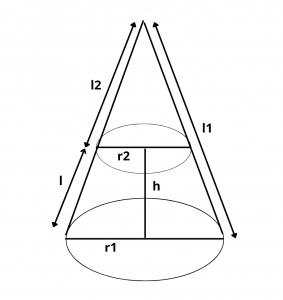# Area of Frustum of Cone – Formula and Derivation

Here you will learn formula for the total surface area and curved surface area of frustum of cone with derivation and examples.

Let’s begin –

## What is Frustum of Cone ?

Frustum of a cone is the solid obtained after removing the upper portion of the cone by a plane parallel to its base. The lower portion is the frustum of a cone.

Height : The perpendicular distance between the aforesaid plane and the base is called the height of the frustum.

## Area of a Frustum of a Cone

### (i) Curved Surface Area (C.S.A) or Lateral Surface Area

C.S.A = $$\pi(r_1 + r_2)l$$

### (ii) Total Surface Area (T.S.A)

T.S.A = $$\pi(r_1 + r_2)l$$ + $$\pi{r_1}^2$$ + $$\pi{r_2}^2$$

where $$l$$ is the slant height of the frustum, $$r_1$$, $$r_2$$ are the radii of the base and the top of frustum of a cone.

Note : Height h of the frustum is given by the relation,

$$l^2$$ = $$h^2$$ + $$(r_1 – r_2)^2$$

### Derivation :

Let $$r_1$$ and $$r_2$$ be the radii of the bottom and top of the frustum respectively and $$l$$ be the slant height of the frustum.Curved Surface area of bigger cone – curved surface area of smaller cone

= $$\pi r_1l_1$$ – $$\pi r_2l_2$$

Since, [ $$l_1\over r_1$$ = $$l_2\over r_2$$ = k ]

= $$\pi[r_1(kr_1) – r_2l(kr_2)]$$

= $$\pi k$$ $$[{r_1}^2 – {r_2}^2]$$

C.S.A = $$\pi k$$$$(r_1 – r_2)$$$$(r_1 + r_2)$$ = $$\pi$$$$(kr_1 – kr_2)$$$$(r_1 + r_2)$$

C.S.A = $$\pi$$ $$(l_1 – l_2)$$$$(r_1 + r_2)$$ = $$\pi l(r_1 + r_2)$$

= (semiperimeter of top and bottom) $$\times$$ (slant height)

Total surface are of frustum = Curved surface area + area of top + area of bottom

T.S.A = $$\pi(r_1 + r_2)l$$ + $$\pi{r_1}^2$$ + $$\pi{r_2}^2$$

Example : A friction clutch is in the form of the frustum of a cone, the diameters of the ends being 8 cm, and 10 cm and length 8 cm. Find its bearing Surface area.

$$r_1$$ = $$10\over 2$$ = 5cm,
$$r_2$$ = $$8\over 2$$ = 4cm
Area of the bearing surface of the cone = $$\pi(r_1 + r_2)l$$
= 3.14(4 + 5) $$\times$$ 8 = 226.08 $$cm^2$$